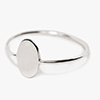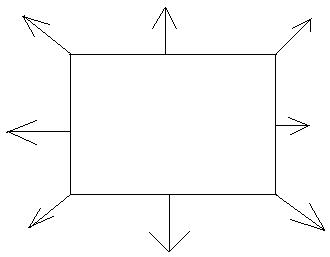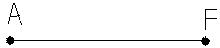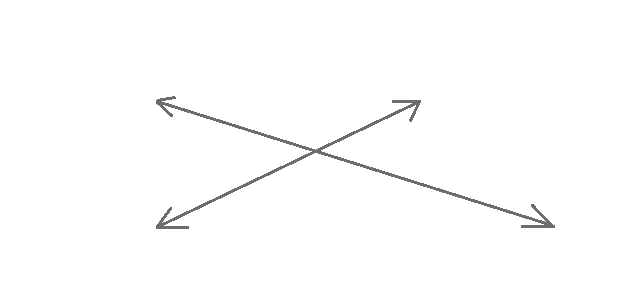Stock last updated on : 23/07/2021##### NEW IN STORES
I think the idea of mixing luxury and mass-market fashion is very modern, very now - no one wears.• No products in the cart.

#### Duksville

Home / Technical / BASIC GEOMETRYBasic geometry is the study of points, lines, angles, surfaces, and solids.The study of this topic starts with an understanding of these. Let’s define them.

Point: A point is a location in space. It is represented by a dot. Point are usually named with a upper case letter. For example, we refer to the following as “point A”Line: A line is a collection of points that extend forever. The following is a line. The two arrows are used to show that it extends forever.We put two points in order to name the line as line AF. However, there are an infinite amount of points. You can also name it line FA.

Line segment: A line segment is part of a line. The following is a segment. A segment has two endpoints. The endpoints in the following segments are A and F. Notice also that the line above has no endpoints.Ray: A ray is a collection of points that begin at one point (an endpoint) and extend forever on one direction. The following is a ray.Angle: Two rays with the same endpoint is an angle. The following is an angle.

Plane: A plane is a flat surface like a piece of paper. It extends in all directions. We can use arrows to show that it extends in all directions forever. The following is a planeParallel lines When two lines never meet in space or on a plane no matter how long we extend them, we say that they are parallel lines The following lines are parallel.Intersecting lines: When lines meet in space or on a plane, we say that they are intersecting linesThe following are intersecting lines.Vertex: The point where two rays meet is called a vertex. In the angle above, point A is a vertex.

[]